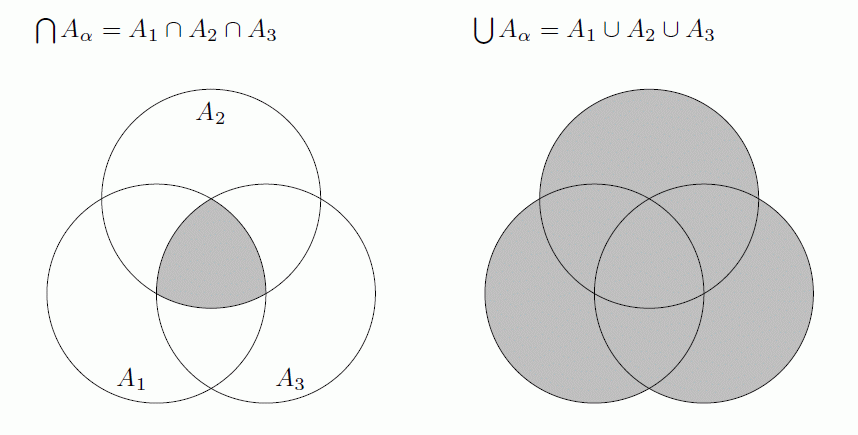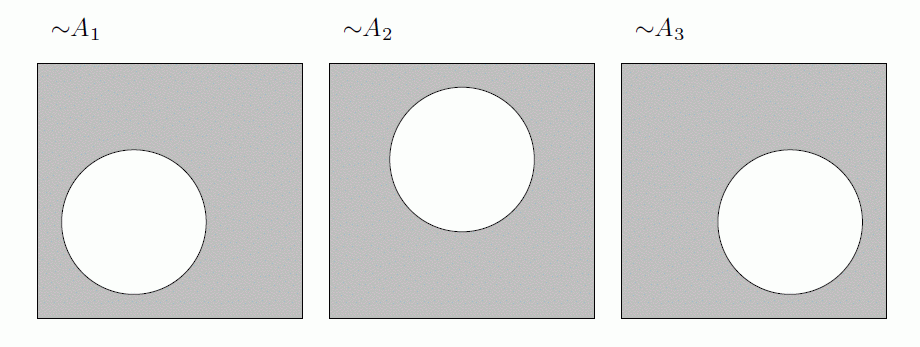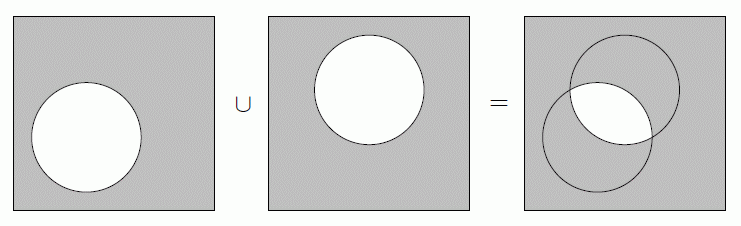DI Management Home > Mathematics > De Morgan's laws explained in graphical form

#De Morgan's laws explained in graphical form

This page explains de Morgan's laws, two important results in set theory concerning complementation, using Venn diagrams.

## The simple form for two sets A and B

For two subsets $A$ and $B$ of a universe $X$, where ${\sim}A$ denotes the complement of $A$ with respect to $X$, de Morgan's two laws state that

\begin{align} {\sim}(A \cup B) = ({\sim}A) \cap ({\sim}B) \tag{1} \\ {\sim}(A \cap B) = ({\sim}A) \cup ({\sim}B) \tag{2} \end{align}

In words, these say that

1. the complement of the union of two sets is equal to the intersection of the complement of each of the sets; and
2. the complement of the intersection of two sets is equal to the union of the complement of each of the sets.

## The general form for a family of sets $A_\alpha$

Using the more general notation where $\bigcup A_\alpha$ denotes the union of a family of sets $\bigcup A_\alpha = A_1 \cup A_2 \cup A_3 \cup \ldots$; and $\bigcap A_\alpha$ denotes the intersection $\bigcap A_\alpha = A_1 \cap A_2 \cap A_3 \cap \ldots$, de Morgan's laws become

\begin{align} {\sim}\bigcup A_\alpha = \bigcap({\sim}A_\alpha) \tag{1a} \\ {\sim}\bigcap A_\alpha = \bigcup({\sim}A_\alpha) \tag{2a} \end{align}

If this notation confuses you, then just go back to the simple form for two sets $A$ and $B$ and substitute $A_1 \cup A_2 \cup A_3 \cup \ldots$ for $A \cup B$; and $A_1 \cap A_2 \cap A_3 \cap \ldots$ for $A \cap B$. In words, replace "two sets" with "family of sets".

## Consider three sets, $A_1, A_2, A_3$

As a simple example, consider the intersection and union of three sets $A_1, A_2, A_3$:The complements of these in the universe $X$ are the shaded bits:Now consider the complements of the individual sets. Think of these as "holes" in the universe $X$. The set ${\sim}A_\alpha$ is the material left outside the hole - shown shaded.Note that the union of two of these complements (the "material" bits) isand that the intersection of two of these complements isSo the union of all the complements $\bigcup({\sim}A_\alpha)$ is everything except for the intersection of the originals $A_\alpha$. Similarly, the intersection of all the complements $\bigcap({\sim}A_\alpha)$ is the "material" left over outside the holes.As a check, note that the intersection (the diagram on the right) is smaller than the union, as expected (remember we are looking at the shaded parts, not the holes).

From these diagrams we can see the results ${\sim}\bigcup A_\alpha = \bigcap({\sim}A_\alpha)$ and ${\sim}\bigcap A_\alpha = \bigcup({\sim}A_\alpha)$.

## Proofs

We use the principle that two sets $X$ and $Y$ are equal if $x \in X$ is equivalent to $x \in Y$. Observe that $x \in {\sim}S$ is equivalent to $x \notin S$.

\begin{align} x \in {\sim}\bigcup A_\alpha &\iff x \notin \bigcup A_\alpha \\ &\iff x \notin A_\alpha \text{ for any } \alpha \\ &\iff x \in {\sim} A_\alpha \text{ for all } \alpha \\ & \iff x \in \bigcap({\sim} A_\alpha). &&\square \\ x \in {\sim}\bigcap A_\alpha &\iff x \notin \bigcap A_\alpha \\ &\iff x \notin A_\alpha \text{ for some } \alpha \\ &\iff x \in {\sim} A_\alpha \text{ for some } \alpha \\ & \iff x \in \bigcup({\sim} A_\alpha). &&\square \end{align}

In the simpler case, here is the proof rewritten for the case of $\alpha = 2$, where $A_1= A$ and $A_2 = B$.

\begin{align} x \in {\sim}(A \cup B) &\iff x \notin A \cup B \\ &\iff x \notin A \text{ and } x\notin B \\ &\iff x \in {\sim} A \text{ and } x \in {\sim} B \\ & \iff x \in {\sim} A \cap {\sim} B. &&\square \\ x \in {\sim}(A \cap B) &\iff x \notin A \cap B \\ &\iff x \notin A \text{ or } x\notin B \\ &\iff x \in {\sim} A \text{ or } x \in {\sim} B \\ & \iff x \in {\sim} A \cup {\sim} B. &&\square \end{align}

## A Joke